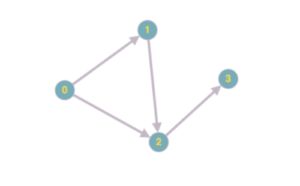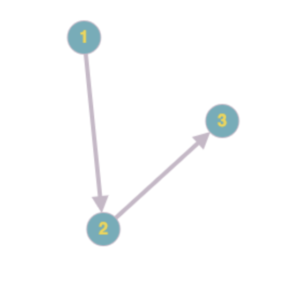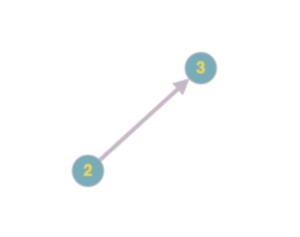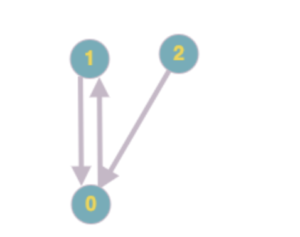## [Leetcode] Binary Tree Right Side View

Given a binary tree, imagine yourself standing on the right side of it, return the values of the nodes you can see ordered from top to bottom.

Example:

```Input: [1,2,3,null,5,null,4] Output: [1, 3, 4] Explanation:   1 <--- / \ 2 3 <--- \ \ 5 4 <---```
• Perform a breath first traversal of the tree also called a level order traversal.
• Before you start processing any level, add the last node from your Q/list to the return list. That’s the node the user would see from the right side.
```/** * Definition for a binary tree node. * public class TreeNode { * int val; * TreeNode left; * TreeNode right; * TreeNode(int x) { val = x; } * } */ class Solution { public List<Integer> rightSideView(TreeNode root) { List<Integer> ret = new ArrayList<Integer>(); LinkedList<TreeNode> q = new LinkedList<TreeNode>(); if ( root != null ) q.add(root); while ( q.size() > 0 ) { int levelSize = q.size(); ret.add(q.getLast().val); for ( int i = 0 ; i < levelSize ; i++ ) { TreeNode curr = q.remove(0); if ( curr.left != null ) q.add(curr.left); if ( curr.right != null ) q.add(curr.right); } } return ret; } }```

## [Leetcode] One Edit Distance

If you’ve solved the edit distance dynamic programming problem, this problem should be straightforward.

1. Make sure to return false if both strings are equal. The problem asks us to return true only if they’re one edit distance apart! Proceed if they’re unequal.
2. Use two pointers set at the end of each string. Find the ‘first’ character thats a mismatch. Keep in mind to stop if either of the pointers reach the end. Even if you exhaust one of the strings and have remaining characters left in the other, its still a mismatch!
3. Once we’ve found ‘one’ mismatch, we need to simply check if inserting, deleting or replacing a character gives us an equal string. For checking this we create a checkEquals() method.
4. checkEquals() method simply checks if two (sub) strings are exactly equal! We’ll pass both the strings and ending indexes i and j to this method. Return whatever checkEquals() returns.
```class Solution { public boolean isOneEditDistance(String s, String t) {   if ( s.equals(t) ) return false;   int i = s.length() - 1; int j = t.length() - 1;   while ( i >= 0 && j >= 0 ) { if ( s.charAt(i) != t.charAt(j) ) break; i--; j--; }   // check if insert, delete or replace returns a valid string! return checkEquals(s, t, i-1, j) || checkEquals(s, t, i, j-1) || checkEquals(s, t, i-1, j-1); }   public boolean checkEquals(String s, String t, int i, int j) { if ( i != j ) return false;   while ( i >= 0 ) { if ( s.charAt(i) != t.charAt(j) ) return false; i--; j--; }   return true; } }```

## [Hackerrank] Pairs

You will be given an array of integers and a target value. Determine the number of pairs of array elements that have a difference equal to a target value.

• Add all elements to a Set
• To figure if an element arr[i] and some X make a pair, we check if
(arr[i] – Target) is in Set

why?
arr[i] – X = Target
X = arr[i] – Target

``` // Complete the pairs function below. static int pairs(int k, int[] arr) { Set<Integer> arrSet = new HashSet<Integer>(); for ( int i = 0 ; i < arr.length ; i++ ) arrSet.add(arr[i]);   int ret = 0; for ( int i = 0 ; i < arr.length ; i++ ) if (arrSet.contains(arr[i]-k)) ret++; return ret; }```

## [Leetcode] Unique Paths II

• ```Total paths ending at [i, j] are Total paths ending at [i+1, j] + Total paths ending at [i, j+1]```
• Typically we would keep track of the path we’re on (so as to not go around in circles). However, in this case since we can only move down or right, there is no way we can circle back to a visited cell.
• Make sure you check for obstacle (and return 0) before checking for end of grid. That way you’ll correctly return 0 for test-case [].
```class Solution { public int uniquePathsWithObstacles(int[][] obstacleGrid) { return uniquePathsWithObstacles(obstacleGrid, 0, 0, new HashMap<String,Integer>()); }   public int uniquePathsWithObstacles(int[][] obstacleGrid, int i, int j, Map<String,Integer> memoized) {   if ( i >= obstacleGrid.length || j >= obstacleGrid.length ) return 0;   if ( obstacleGrid[i][j] == 1 ) return 0;   if ( i == obstacleGrid.length-1 && j == obstacleGrid.length-1 ) return 1;   String key = String.valueOf(i) + "," + String.valueOf(j); if (memoized.containsKey(key)) return memoized.get(key);   int totalPaths = uniquePathsWithObstacles(obstacleGrid, i+1, j, memoized) + uniquePathsWithObstacles(obstacleGrid, i, j+1, memoized); memoized.put(key, totalPaths);   return totalPaths; } }```

## [Leetcode] Course Schedule II

What is topological sort? You simply keep picking nodes with no incoming edges (or with in-order 0).No valid solution? You might encounter situations where none of the nodes (yet to be processed) have an inorder of 0. This means that the graph does not have a valid topological sort (and we need to account for that). Here is an example. After picking ‘2’, you’re stuck with this 1-0, 0-1 cycle where inorder’s for both nodes are 1.Basic Idea:

• Create a dependency graph using the dependencies. The topological sort of this graph is your solution. Represent your graph using a HashMap (node_id -> [ child_id1, child_id2, .. child_idN ]).
• Use a Q to keep track of nodes with inorder 0. Don’t loop through all nodes to check for nodes with inorder 0 (except for the first time). Remember, at every step the only nodes that have a potential to have inorder 0 are the children of the last picked node!
• Finally, if you haven’t processed all the nodes (which means there is no valid topological sort) remember to return empty array.
```class Solution { public int[] findOrder(int numCourses, int[][] prerequisites) { Map<Integer, List<Integer>> graph = new HashMap<Integer, List<Integer>>(); int inorders[] = new int[numCourses]; int order[] = new int[numCourses]; int orderIndex = 0;   // build graph for ( int i = 0 ; i < prerequisites.length ; i++ ) { List<Integer> children = graph.getOrDefault(prerequisites[i], new ArrayList<Integer>()); children.add(prerequisites[i]); graph.put(prerequisites[i], children); inorders[prerequisites[i]]++; }   // have q keep track of all nodes with inorder = 0 // we will keep adding nodes to this q as and when thier inorder get to 0 Queue<Integer> q = new LinkedList<Integer>(); for ( int i = 0 ; i < inorders.length ; i++ ) { if (inorders[i] == 0) { q.add(i); } }   // pick nodes with zero indegree and add to list // make sure we update (decrement) indegrees of child nodes while ( !q.isEmpty() ) { int nextNode = q.remove(); List<Integer> children = graph.get(nextNode); if ( children != null ) { for ( Integer child : children ) { inorders[child]--; if (inorders[child] == 0) { q.add(child); } } } order[orderIndex++] = nextNode; }   // For cases where there are cycles in graphs we wont get a topological sort for all the nodes. T his is a way to detect that! // Examples: [[0,1],[1,0]], [[1,0],[1,2],[0,1]] if ( orderIndex < numCourses ) return new int;   return order; } }```

## [Leetcode] Container With Most Water

```class Solution { public int maxArea(int[] height) { int i = 0 ; int j = height.length-1; int max = Integer.MIN_VALUE;   while ( i < j ) { int currwidth = j-i; int currheight = Math.min(height[i], height[j]); max = Math.max(max, currwidth*currheight);   if ( height[i] < height[j] ) i++; else j--; }   return max; } }```